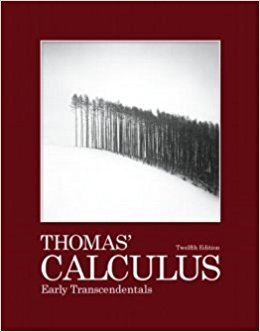# Solutions for Chapter 9: First-Order Differential Equations## Full solutions for Thomas' Calculus Early Transcendentals | 12th Edition

ISBN: 9780321588760Solutions for Chapter 9: First-Order Differential Equations

Solutions for Chapter 9
4 5 0 324 Reviews
10
2
##### ISBN: 9780321588760

This textbook survival guide was created for the textbook: Thomas' Calculus Early Transcendentals, edition: 12. Chapter 9: First-Order Differential Equations includes 36 full step-by-step solutions. This expansive textbook survival guide covers the following chapters and their solutions. Since 36 problems in chapter 9: First-Order Differential Equations have been answered, more than 4123 students have viewed full step-by-step solutions from this chapter. Thomas' Calculus Early Transcendentals was written by and is associated to the ISBN: 9780321588760.

Key Calculus Terms and definitions covered in this textbook
• Angle

Union of two rays with a common endpoint (the vertex). The beginning ray (the initial side) can be rotated about its endpoint to obtain the final position (the terminal side)

• Associative properties

a + (b + c) = (a + b) + c, a(bc) = (ab)c.

• Blind experiment

An experiment in which subjects do not know if they have been given an active treatment or a placebo

• Cardioid

A limaçon whose polar equation is r = a ± a sin ?, or r = a ± a cos ?, where a > 0.

• Convenience sample

A sample that sacrifices randomness for convenience

• Gaussian curve

See Normal curve.

• Jump discontinuity at x a

limx:a - ƒ1x2 and limx:a + ƒ1x2 exist but are not equal

• Multiplicative inverse of a matrix

See Inverse of a matrix

• nth root

See Principal nth root

• Numerical model

A model determined by analyzing numbers or data in order to gain insight into a phenomenon, p. 64.

• Perpendicular lines

Two lines that are at right angles to each other

A procedure for fitting a quadratic function to a set of data.

• Reciprocal function

The function ƒ(x) = 1x

• Residual

The difference y1 - (ax 1 + b), where (x1, y1)is a point in a scatter plot and y = ax + b is a line that fits the set of data.

• Semiperimeter of a triangle

One-half of the sum of the lengths of the sides of a triangle.

• Solve by elimination or substitution

Methods for solving systems of linear equations.

• Square matrix

A matrix whose number of rows equals the number of columns.

• Transitive property

If a = b and b = c , then a = c. Similar properties hold for the inequality symbols <, >, ?, ?.

• Translation

See Horizontal translation, Vertical translation.

• x-intercept

A point that lies on both the graph and the x-axis,.

×

I don't want to reset my password

Need help? Contact support

Need an Account? Is not associated with an account
We're here to help